• 详解VBA单元格、工作表、工作簿各种表示、方法，注意事项。
VBA单元格、工作表、工作簿

一、单元格
注意：
单元格不仅包括值，还包括格式等信息，虽然很多时候结果是等同的，为避免出错，如果只想引用单元格的值，使用.value方法，尤其在使用字典时，比如：dic(range(“a1”).value) = range(“b1”).value；赋值：range(“a1”).value = 100直接输入单元格，表示的是当前激活工作表的单元格，如果想表示非激活工作表单元格，需要在单元格前面加上工作表对象，比如sheets(“sheet_name”).range(“a1”)；这里工作表也是一样的，默认为激活工作簿下的工作表，如需表示其他工作簿工作表，需要在前面加上工作簿对象；未避免出错，一般我们都会设置工作簿对象，逐级引用下面的单元格对象。比如：set wb = thisworkbook
with wb.worksheets("sheet_name")
.range("a1").value = "a1"
.cells(2,1).value = "a2"
[a3].value = "a3"
end with

1. range表示
1.1 单个单元格：
range("a1")
'或者
range("ab200")

1.2. 范围：
1.2.1. 指定范围
'如果指向同一个工作表，这三个是等价的，表示a1:b10范围
range("a1:b10")
range("a1","b10")
range(range("a1"),range("b10"))

1.2.2. 行或者列
'行表示：
range("a1").entirerow
range("1:1")
range("2:2,5:5")
rows(1)
rows("1:1")
'列表示：
range("a:a")
columns("a:a")
columns(1)
range("a1").entirecolumn

1.2.3. 当前区域和使用区域
sheet1.usedrange	'使用区域
sheet1.range("a1").currentregion		'单元格a1所在连续区域

1.2.4. 交集和合集
intersect(range("a1:a10"),range("2:3"))		'该交集结果：range("a2:a3")
rg = range("a1")
rng = range("a2")
set rng = union(rng,rg)		'union单元格取并集，该并集结果：range("a1:a2")；注意：对象要使用set赋值。

1.2.5 向range传入excel单元格自定义名称
'还可以向range传入excel单元格自定义名称
'是的，可以向多个单元格赋值同一个数值
range("input") = 1

运行效果如下图：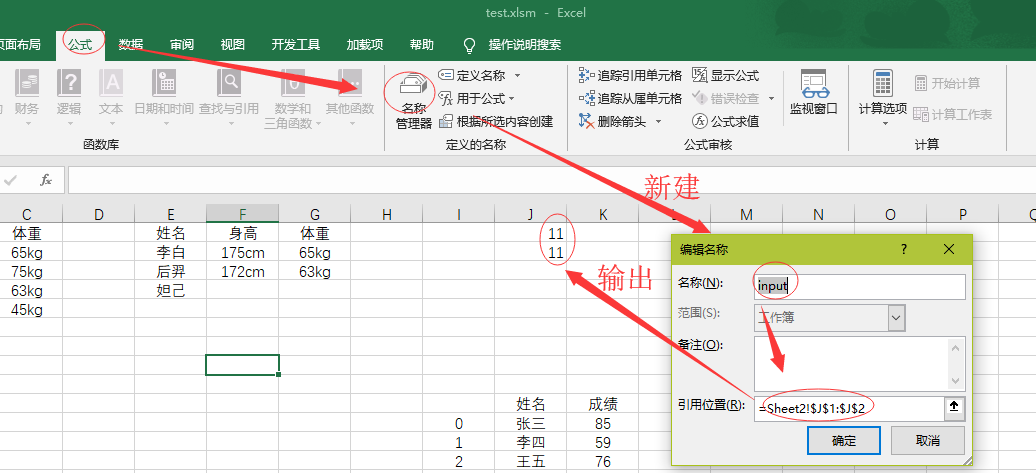1.2.6 定位
range("a1:b10").specialcells(xlCellTypeBlanks)		'定位空白单元格
sheets(sheet_name).specialcells(xlcelltypevisible)		'定位可见单元格

specialcells更多参数请参考VBA帮助手册。
其中，entirerow和entirecolumn,currentregion,union,specialcells同样适用于[]和cells表示使用，下面不再重复赘述。
2. 其他表示方法
2.1 cells单个单元格
'都表示a1单元格
cells(1,1)
cells(1,"a")
'下面这种表示，range("a2:b6")一共有10个单元格，如果想cells传入一个数值，表示从左上到右下的位置，下面表示为range("a4")单元格
range("a2:b6").cells(5)
'表示range("a5")单元格
rang("a2:b6").cells(4,1)
''Range("a2:b6")只有10个单元格，cells传入参数实际是从单元格左上角开始计算的。可以忽略区域大小
Range("a2:b6").Cells(12) = "第12个单元格"

range使用cells方法效果如图：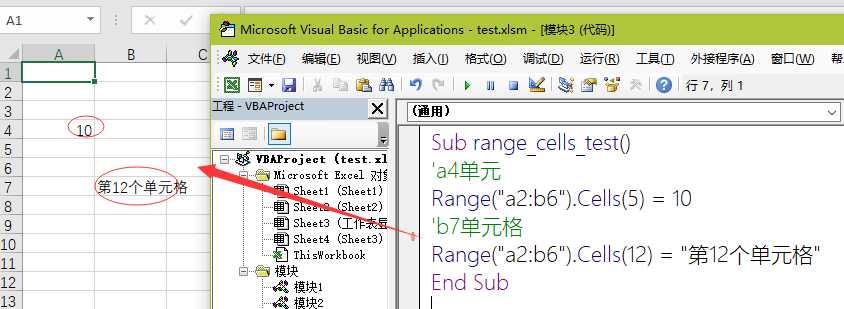2.2 []表示方法
[a1]	'range("a1")
[a1:b10]	'range("a1:b10")

2.3 活动单元格 ActiveCell，如果在select选中单元格情况下，也可以使用selection active也适用于工作表，工作表，图表等，表示活动对象。比如活动sheet：activesheet
3. end方法
等同于excel中“ctrl”+方向键定位。range(“b3”).end(xlup) 向上定位：range(“b1”) end可以传入参数’上’:‘xlup’,‘下’:‘xldown’,‘左’:‘xltoleft’,‘右’:‘xltoright’ 也可以传入数字1，2，3，4分别代表上下左右。 注意：end只能在连续单元格跳转到最后一个单元格，range(“b10”).end(xlup)，如果b5单元格为空，只会跳转到b6单元格，跟excel一样的。
4. 格式
4.1 判断单元格是否包含批注
If Range("A1").Comment Is Nothing Then
'插入"hello"批注：

4.2 单元格是否为公式单元格
if range("a1").hasformula = True then

这点可以结合公式填充语句filldown（excel鼠标公式单元格右下角双击公式填充）一起使用，先判断单元格是否为公式单元格，再调用filldown。如下代码：
Sub formula_filldown()
dim i ,max_row,max_col as long
max_col = activesheet.[a1].end(xltoright).column
max_row = activesheet.usedrange.rows.count
'假设第一行是标题，第二行是内容
for i = 1 to max_col
'判断第二行单元格是否包含公式，有公式自动填充
if cells(2,i).hasformula then range(cells(2,i),cells(max_row,i)).filldown
next
End Sub

4.3 是否为合并单元格
range("a1").mergecells = true
'合并单元格
range("a1:b1").merge

4.4 格式刷&选择性粘贴
格式刷
sub paste_formats()
Range("a6:at6").Copy
Range("a7:at7").PasteSpecial Paste:=xlPasteFormats
end sub

选择性粘贴为值
With ActiveSheet.UsedRange
.Copy
.PasteSpecial Paste:=xlPasteValues
'复制后要清空剪切板，不然再操作复制，会出错。
Application.CutCopyMode = False
End With

5. 排序
key表示排序字段，第一个有值的单元格，非标题。order排序方法，升序：xlAscending，降序：xlDescending；可以设置多个排序字段，key-order依次写就可以了。
Sub sort()
Range("a2:b12").sort key1:=Range("a2"), order1:=xlDescending, _
key2:=Range("b2"), order2:=xlAscending
End Sub

6. 筛选
Sheet1.UsedRange.AutoFilter field(筛选的列),criteria1(筛选条件，可以使用比较运算符),operator(第二筛选条件),visibledropdown(是否显示箭头True：显示) 范围也可以指定为数组array，比如： range(“a1”).currentregion.autofilter field:=3,criteria1:=array(…) 示例图片：Sub aa()
'筛选地区-广东
Sheet1.UsedRange.AutoFilter field:=1, Criteria1:="广东"
'筛选水果-苹果
Sheet1.UsedRange.AutoFilter field:=2, Criteria1:="苹果"
'筛选销量-大于1
Sheet1.UsedRange.AutoFilter field:=3, Criteria1:=">1"
End Sub

结果如下图：7. 隐藏行或列
'隐藏C和E列
Range("C:C,E:E").EntireColumn.Hidden = True

'取消行列隐藏
Cells.EntireColumn.Hidden = False
Cells.EntireRow.Hidden = False

8. 删除与插入
'clear是清除内容与格式

'清除工作表中的内容。
cells.clear

'或者也可以这样
sheet1.usedrange.clear

'如果你只想清楚单元格内容的话，使用.clearcontents方法
sheet1.range("a1:b100").clearcontents

'删除行
Worksheets("Sheet1").Rows(3).Delete	'删除第三行

'插入行
Worksheets("Sheet1").Rows(3).Insert

'插入列
Columns("b").Insert

9. 自动调整列宽
Range("a1:h1").EntireColumn.AutoFit

10. 单元格范围与偏移使用
适用于单元格对象
'使用resize表示单元格范围
'如果只传入一个参数的话，表示以单元格为起点到向下第10个单元格的连续单元格区域。这里相当于range("a1:a10")
Range("a1").Resize(10).Value = 1
'传入两个参数，第一个以单元格为起点的区域单元格行数，第二个参数以单元格为起点的区域单元格列数
Range("b1").Resize(2, 3).Value = 2

'offset是偏移量，类似excel的offset函数，第一个参数上下（负正）偏移，第二个参数左右（负正）偏移，如果前面是一个单元格对象，参数是可以传入负数的。
Range("b4").Offset(2).Value = 3
Range("b4").Offset(2, 2).Value = 4
Range("b4:c5").Offset(3, 2).Value = 5
Range("c9").Offset(1, -1).Value = 6

使用效果图下图：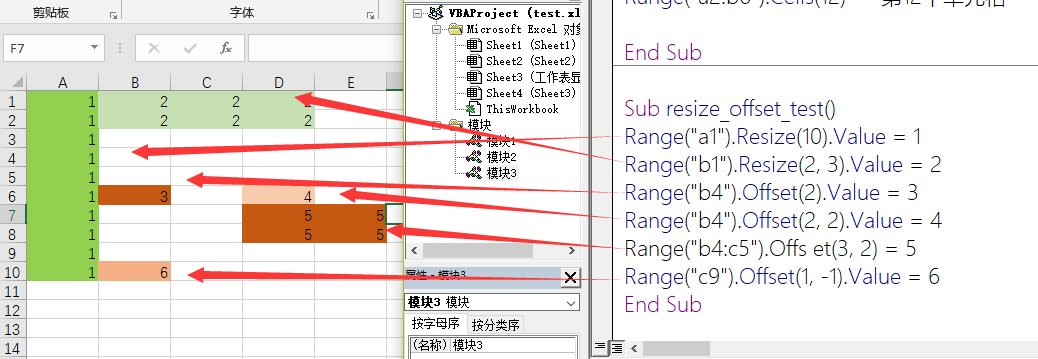二、工作表-工作簿
1. 工作表&工作簿表示
1.1 工作表表示 如下图片选中，工作表一共有三种表示。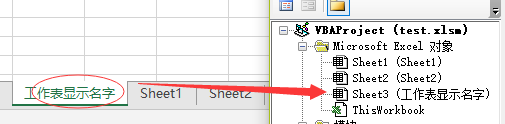使用工作表隐式名字：sheet3使用工作表显示名字：sheets(“工作表显示名字”)，通常情况下，显示名字跟隐式名字是一样的，如果是一样的，也可以这么表示：sheets(“sheet3”)使用索引：sheets(1) 根据工作表在excel中从左到右的位置，该工作表为第一个。当然也可以使用活动工作表：activesheet，active跟单元格，工作簿等是一样适用的。使用父对象：range("a1").parent 1.2 工作簿表示
这个工作簿：thisworkbook，写代码所在工作簿活动工作簿：activeworkbook使用索引：workbooks(1) 同工作表使用工作簿名字：workbooks(“工作簿名字”)使用父对象：range("a1").parent.parent
1.3 工作簿设置对象 一般我们会为工作簿设置对象，方便引用，如果存在多个对象，这样就不会混乱。 set wb = workbooks.open(“需要打开工作簿完整路径”)
2. 常用语句
2.1 工作表
2.1.1 新建工作表
ThisWorkbook.Sheets.Add Before:=Worksheets(1)
activesheet.name = "新建工作表命名"

或者可以直接这样：
Sheets.Add.Name = "新建工作表命名"

2.1.2. 取消筛选 如果工作当前有筛选的话，取消筛选。
If Sheets("明细").AutoFilterMode = True Then Sheets("明细").AutoFilterMode = False

2.1.3. 工作表隐藏 如果你不想某些工作表展示的话，可以这么操作，当visible参数为2时（深度隐藏），工作表鼠标右击操作是无法显示工作簿的； (-1：显示；0：隐藏；2：深度隐藏)
'深度隐藏sheet1工作表
sheet1.visible = -2

2.1.4. 删除工作表
sheet1.detete	删除工作表

2.1.5. 取消当前工作表所有的超链接
Cells.Hyperlinks.DeleteCells.Hyperlinks.Delete

2.1.6 工作表复制（复制到原工作簿或新工作簿）
Sub sheet_copy_to_new_workbook()
Dim new_wb As Workbook
Dim st
dim i as Byte
With ThisWorkbook
'复制工作表，如果没有指定after（位置）参数，就会自动创建一个新的工作簿,复制工作表到新创建的工作簿
.Sheets(1).copy
'设置新创建工作簿对象
Set new_wb = ActiveWorkbook
For i = 2 To .Sheets.Count
.Sheets(i).copy new_wb
'如果是下面这样写的话，是在原来工作簿上复制工作表，复制的工作表位置放在最后面。
'.Sheets(i).copy after:=worksheets(sheets.count)
.Sheets(i).copy after:=new_wb.Sheets(new_wb.Sheets.Count)
'也可以给复制的工作表重新命名
ActiveSheet.Name = i
Next
End With
'保存新工作簿到指定路径，命名为“new_workbook.xlsx”
wb.SaveAs "d:/桌面/new_workbook.xlsx"
'这里其实是复制了当前工作簿(复制工作表示例)，如果不想做其他修改，比如修改表名等信息，也可以使用文件复制语法：FileCopy+Name实现文件复制及重命名
'语法如下：
FileCopy "f:\initial.xlsx", "e:\copy_file.xlsx"
Name "e:\copy_file.xlsx" As "e:\new_name.xlsx"
End Sub

2.2 工作簿
2.2.1 打开工作簿,并指定对象
set wb = workbooks.open("d:/Desktop/test.xlsm")

2.2.2 新建工作簿另存
Set wb = Workbooks.Add
'复制这个工作簿“通报”工作表的内容到新工作簿工作表
ThisWorkbook.Sheets("通报").[a1].CurrentRegion.Copy wb.Worksheets("Sheet1").[a1]
'工作簿另存为
wb.saveas "d:/Desktop/新工作簿名字.xlsx"
'关闭工作簿，并且保存,如果后面的参数为False，如果没有事先保存的话，则会丢失修改数据。
wb.close True

2.2.3 保存工作簿
thisworkbook.save
'或者也可以这样
thisworkbook.saved = true

2.2.4 工作簿的名字
thisworkbook.name
'工作簿带路径完整的名字
thisworkbook.fullname

2.2.5 删除工作簿
'kill + fullname,使用kill方法也可以删除其他文件，后面带完整路径。
kill "d:\Desktop\wb_delete.xlsx"

展开全文• 1 单元格的值 Sub x1() Range("b10") = Range("c2").Value Range("b11") = Range("c2").Text Range("b11") = "'" & Range("c2").Formula '获取单元格公式 End Sub 2 单元格的地址 Sub x2() With
1 单元格的值
   Sub x1()
Range("b10") = Range("c2").Value
Range("b11") = Range("c2").Text
Range("b11") = "'" & Range("c2").Formula     '获取单元格的公式
End Sub
2 单元格的地址
    Sub x2()
With Range("b2").CurrentRegion
End With
End Sub
3 单元格的行列信息
 Sub x3()
With Range("b2").CurrentRegion
[b13] = .Row
[b14] = .Rows.Count
[b15] = .Column
[b16] = .Columns.Count
End With
End Sub
4、单元格的格式信息
    Sub x4()
With Range("b2")
[b19] = .Font.Size
[b20] = .Font.ColorIndex
[b21] = .Interior.ColorIndex
[b22] = .Borders.LineStyle
End With
End Sub
5、单元格批注信息
     Sub x5()
[B24] = Range("I2").Comment.Text
End Sub
6 单元格的位置信息
     Sub x6()
With Range("b3")
[b26] = .Top
[b27] = .Left
[b28] = .Height
[b29] = .Width
End With
End Sub
7 单元格的上级信息
    Sub x7()
With Range("b3")
[b31] = .Parent.Name
[b32] = .Parent.Parent.Name
End With
End Sub
8 内容判断
      Sub x8()
With Range("i3")
[b34] = .HasFormula              '是否含有公式
End With
End Sub
展开全文• 本期主要讲讲单元格复制粘贴等操作。... '注意：单元格的格式、公式、批注等也会被复制过去哟 Sheets(1).Range("A1").Copy Sheets(1).Range("B1") End Sub Sub CopyRange2() '复制单元格内容示例2，打开
本期主要讲讲单元格复制粘贴等操作。
一、复制粘贴固定区域的单元格
因为以下内容都是复制单个或者固定区域的单元格内容，就不多说了，备注上面都有说明。
Option Explicit

Sub CopyRange()
'复制单元格内容示例1
'注意：单元格的格式、公式、批注等也会被复制过去哟
Sheets(1).Range("A1").Copy Sheets(1).Range("B1")
End Sub

Sub CopyRange2()
'复制单元格内容示例2，打开了下面2个工作表后，就可以通过工作表的名称进行复制啦
Workbooks("测试表1.xlsx").Sheets(1).Range("A1").Copy _
Workbooks("测试表2.xlsx").Sheets(1).Range("A1")
End Sub
Sub CopyRange3()
'复制单元格内容示例3，和上面几乎一样
Dim rng1 As Range, rng2 As Range
Set rng1 = Workbooks("测试表1.xlsx").Sheets(1).Range("A1")
Set rng2 = Workbooks("测试表2.xlsx").Sheets(1).Range("A1")
rng1.Copy rng2
End Sub
Sub CopyRange4()
'复制单元格内容示例4，复制区域，选择一个单元格进行粘贴
Sheets(1).Range("A1:D5").Copy Sheets(2).Range("A1")
End Sub
Sub MoveRange1()
'移动（剪切）单元格内容示例1。
'注意：单元格的格式、公式、批注等也会被剪切过去哟
'就是把Copy换成了Cut，其他几乎一样
Sheets(1).Range("A1").Cut Sheets(1).Range("B1")
End Sub
二、复制粘贴可变单元格区域
上面主要是讲的特定区域的，下面讲讲会变动的区域。比如一张表新增了一行，复制区域就改变了，有没有什么比较方便的方式解决呢？
加个CurrentRegion就可以实现，用法如下。
Sub CopyCurrentRange()
'复制范围会变动的表格
Sheets(1).Range("A1").CurrentRegion.Copy Sheets(2).Range("A1")
End Sub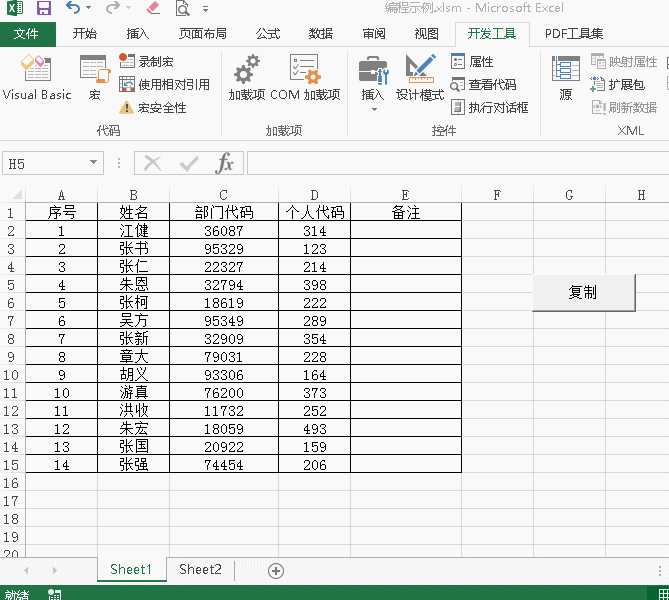从上面的图可以看出，新增了第15行张三点击运行后，仍然能正常复制过去。要复制的表如果有标题、有日期行，或者是筛选后的表格，仍然可以实现复制。
如果要复制的区域是一个表，就可以用以下方式（感觉更复杂了点，好像没啥实际用途）
第一，插入表格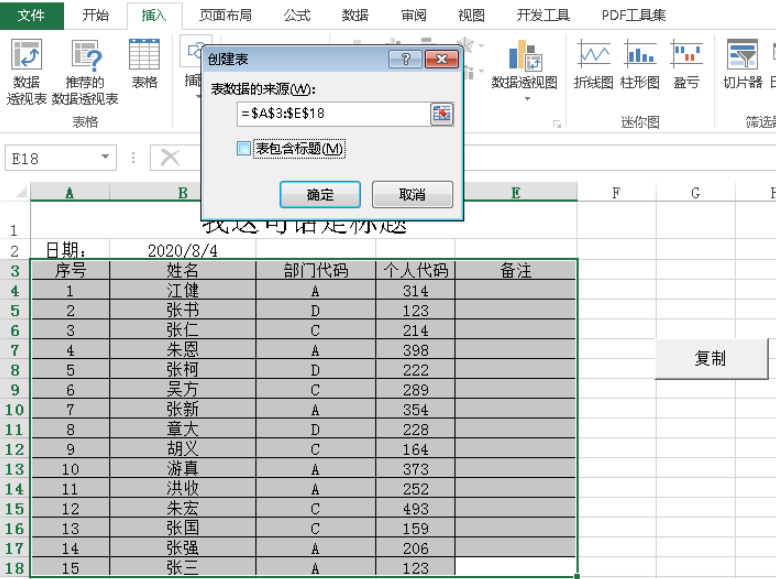第二，重新命名，咱们插入表格之后，在设计——表名称那重命名一下，命名为Table111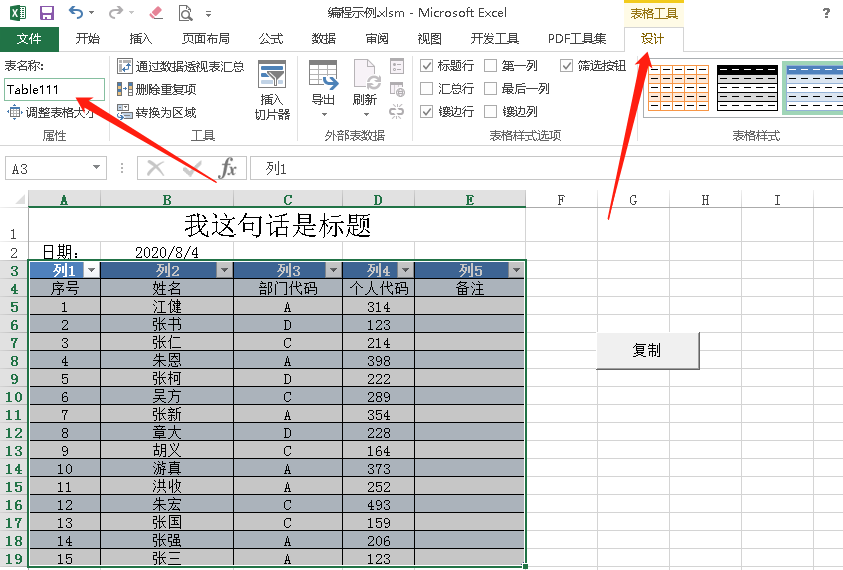第三：这么操作就可以把表复制过去啦。
Sub CopyTable()
Sheets(1).Range("table111").Copy Sheets(2).Range("A1")
End Sub
经过实际使用，发现确实不怎么方便，当然了还有Range的End方法，这个在7月29号的文档里用过。
Sub RangeEnd()
'从A10000往上数，遇到有数据的最下面一行
Debug.Print Sheets(1).Range("A10000").End(xlUp).Row
'从A1往下数，有数据的最下面一行
Debug.Print Sheets(1).Range("A1").End(xlDown).Row
'从Z1往左数，有数据的最右边一列
Debug.Print Sheets(1).Range("Z1").End(xlToLeft).Column
'从A1往右边数，有数据的最右边一列
Debug.Print Sheets(1).Range("A1").End(xlToRight).Column
End Sub
咱们表格范围是A1:D17大小的话，上面结果值分别为17，17，5，5。但是需要注意就是，选定单元格的那一行一列别有空值，不然返回的内容可能是空值附近的单元格哟。
三、调整单元格区域
用Range.Resize重新调整单元格区域，左边的参数是行数，右边的参数是列数。
另外需要注意的是，调整后的区域是从原来区域左上角开始框哟。
比如下面例子开始是框柱B2:D6，重新设置区域后，就从B2往下和往右算行列数。
Sub RangeResize()
Dim rng As Range
Set rng = Range("B2:D6")
Set rng = rng.Resize(8, 5)
End Sub


展开全文• 继续讲讲单元格的操作 一、提示用户输入值 Sub SetValue() Sheets("sheet3").Range("a1") = InputBox("输入一个数字") End Sub 运行后就会弹出个警告框，提示用户输入内容，输入后就会在A1单元格显示了，但是也...
继续讲讲单元格的操作
一、提示用户输入值
Sub SetValue()
Sheets("sheet3").Range("a1") = InputBox("输入一个数字")
End Sub
运行后就会弹出个警告框，提示用户输入内容，输入后就会在A1单元格显示了，但是也存在一个问题，就是取消后呢，原来在A1单元格的内容就会被清空。
所以改良一下，也把“输入数字这个功能再完善一下”。
Sub SetValue()
Dim myInput As Variant
myInput = InputBox("输入一个数字")
If myInput <> "" Then
If IsNumeric(myInput) Then
Sheets("sheet3").Range("a1") = myInput
Else
MsgBox "输入的不是数字"
End If
Else
MsgBox "输入的内容为空值"
End If
End Sub

在判断是否是数字上，如果用WorksheetFunction.IsNumber判断可能会出错。用户在输入123后，WorksheetFunction.IsNumber仍然会识别成文本格式。经过资料查询，解释是这样的：
WorksheetFunction.IsNumber：函数中的数值参数是无法转换的。例如，大部分其他函式中其中一个数字是必要，文字值19会转换的数字19。不过，在公式中ISNUMBER（“19”）、19不会转换文字值，且IsNumber函数会传回False。
IsNumeric：如果在整个表达式被视为一个数字就为真，否则，它会传回False。如果_表达式_为日期表达式IsNumeric会传回False。
所以咱们就知道为什么IsNumeric用来判断用户输入是否数字可以，而WorksheetFunction.IsNumber不行啦。

二、在空值输入内容
在空值输入内容的第一步当然是确定空值的位置啦，运行后跳转到空值的地方进行输入。我这里主要用到昨天的Range.End(xldown)来确定下一行的空值。
Sub SetBlankValue()
Dim rowNum As Integer
rowNum = Range("a1").End(xlDown).Row + 1
Range("a" & rowNum).Select
End Sub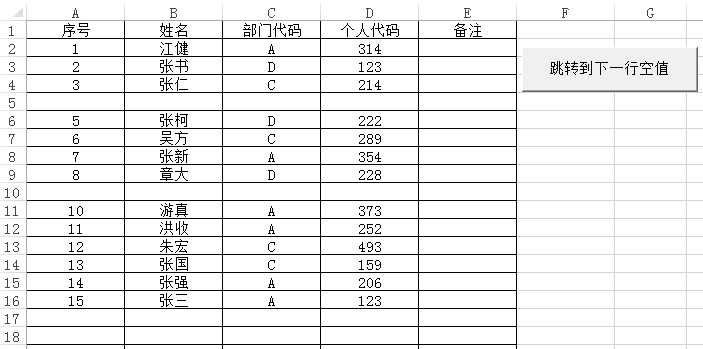是不是很简单啦。

三、计算选中单元格 数量
MsgBox Selection.Count
框柱单元格后运行就出来了，一句话完事儿~
如果要有选择多个选区，想知道多个选取的数量，用以下方式
MsgBox Selection.Areas.Count

四、有效遍历选区
有效遍历选区一般是对选区内有值的进行遍历操作，不然大量空值存在就很浪费时间，下面是对用户框住的内容进行判断，小于0的就成黄色，大于等于0的就蓝色。
Sub BianLi()
Dim cell As Range
If TypeName(Selection) <> "Range" Then Exit Sub
Application.ScreenUpdating = False
For Each cell In Selection
If cell.Value < 0 Then
cell.Interior.Color = RGB(255, 255, 0)
Else
cell.Interior.Color = RGB(0, 255, 255)
End If
Next
End Sub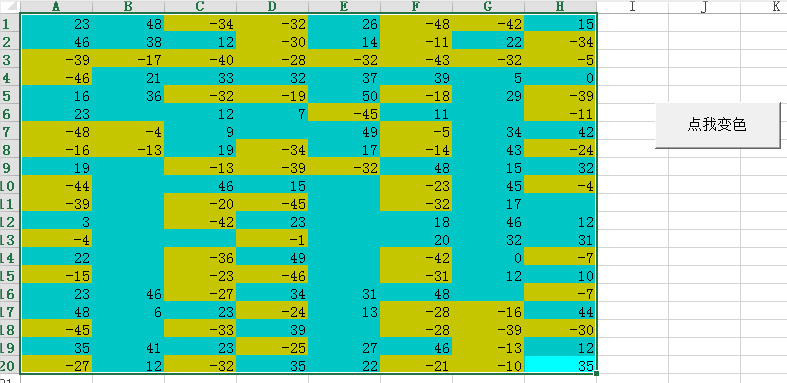框选后确实变色了，但是呢如果选中整行整列的话，会比较慢，工作表有个叫UsedRange的方法，官方解释【返回一个**Range** 对象, 该对象代表指定工作表上的所用区域。】也就是咱们用了多少单元格意思。输出个已使用单元格数量试试
MsgBox (Sheets(1).UsedRange.Count)
然后我们就可以根据用户框住的区域和这个UsedRange区域进行交叉匹配，匹配到了的说明有值，需要进行运算
Sub BianLi2()
Dim cell As Range, rng As Range
If TypeName(Selection) <> "Range" Then Exit Sub
Application.ScreenUpdating = False
Set rng = Application.Intersect(Selection, ActiveSheet.UsedRange)
For Each cell In rng
If cell.Value < 0 Then
cell.Interior.Color = RGB(255, 255, 0)
Else
cell.Interior.Color = RGB(0, 255, 255)
End If
Next
End Sub
结果和上图相似，但是速度会稍稍快了点，但是也带来一个问题，如果用户点了空白区域，既没有内容，也没有使用，所以可能会报错哟，所以还需要改进一下。
此刻需要用表达式。Range.specialcells(Type, Value)来区分一下，该函数返回一个Range对象, 该对象代表与指定类型和值匹配的所有单元格。第一个参数是要包含的单元格，必填；第二个参数是可选的，写上要返回哪些类型的单元格
所以咱们把有值的Range用这个函数拆成2部分，数字的，就做判断，不是数字的，就不管了
Sub BianLi3()
Dim numCells As Range, cell As Range
If TypeName(Selection) <> "Range" Then Exit Sub
Application.ScreenUpdating = False

'xlConstants 意思是单元格是值，xlNumbers意思就是数字
Set numCells = Selection.SpecialCells(xlConstants, xlNumbers)

For Each cell In numCells
If cell.Value < 0 Then
cell.Interior.Color = RGB(255, 255, 0)
Else
cell.Interior.Color = RGB(0, 255, 255)
End If
Next
End Sub


展开全文• 利用VBA计算出总成绩，但是要由公式计算得出，也就是说，VBA运行完毕后，单元格C2内是有公式的。 VBA代码 以上特别需要注意的是什么呢？就是引号，输入公式本身和在excel内自己填写公式没有区别，重点是要用引号...
• 那么，如何在VBA中向单元格中输入公式呢？看到别人的培训资料里已有所总结，这里借花献佛一下。代码：Option Explicit '一、在单元格中输入公式 '1、用VBA单元格中输入普通公式 Sub t1() Range("d2"...
• 我正在使用VBA代码在Excel 2016工作表中插入新行，然后将公式从上面的行复制到新行，这样我就不必手动插入它们 . 不幸的是，复制公式后，插入公式单元格受到保护 . 这带来的缺点是，如果我犯了错误，我就无法删除...
• Excel的公式可谓是其中的精华，但是发布文件时，有时需要将工作表单元格中的公式转换为静态结果，在Excel中进行操作使用选择性粘贴为数值将可以实现。 如果有很多工作表需要进行这种转换，逐个去处理和操作，也是很...静态化 Excel 数据转换
• 利用Formula属性给单元格输入公式 Formula属性和Value属性的联系与区别 利用FormulaR1C1属性给单元格输入公式 R1C1引用样式的规则 (1)“绝对引用”方式 (2)“相对引用”方式 (3)“混合引用”方式 通过代码...
• 1.单元格取值赋值 PrivateSubCommandButton1_Click() Range("A1")="111" Range("A2").Value="2222" Range("A10")=Range("A1") Range("A11")=Cells(2,1).Value Range("A12")=Cells(2,1) Range("...
• 最近的项目中，用到了很多Excel的VBA宏功能，用户的新需求也有很多需要用VBA宏来实现。为满足业务需求， 自己搜索网上的相关资料，尝试不同的解决方法，最终解决了用户的需求，在此记录下来，做一个总结，也希望能...
• 先看一个简单的循环程序，VBA从数据库中读取数据后，需要在最后一个单元格输入求和公式： lineno = [A65536].End(xlUp).Row For i = 3 To lineno Cells(i, 9).FormulaR1C1 = "=RC[-3]+RC[-2]+RC[-1]" Next i 公式...
• 参数： Code：查询的内容 Zone：查询的区域 ColumnIndex：指定返回列的序号 Splitor：行分隔符 '连接字符串，类似PHONETIC，但是可以添加分隔符 FunctionConnectString(ZoneAsrange,SplitorAsString) ...
• sub CellFormulaIsWrong() if IsError(range("a1").value) = true then msgbox "a1 单元格错误类型为:" & range("a1").text ... MsgBox "a1 单元格公式结果为:" & range("a1").value end if End Subexcel
• Function ISFORMULA(ByVal rg As Object) As Variant Dim temp As Variant Dim i As Integer, j As Integer temp = rg.Formula If IsArray(temp) Then For i = 1 To UBound...
• 我们如何对单元格进行公式赋值 dim bookname as string dim sheetname as string dim rangename as string Workbooks(bookname).Worksheets(sheetname).Range(rangename).Formula = "=sum("E1")" 对单元格里面...
• 平时工作中经常会用到excel的功能, 难免有一些操作不能批量处理, 比如说某一列有100w的数据需要合并单元格, 手动去点的话, 费时费力, 又不能保证准确...请出公式大神 2.vba 3.vbs 论效率和学习成本来说, vba是最佳方案.
• 当需要读取单元格公式并修改公式内容时，通常要用到FORMULATEXT函数，以字符串的形式返回公式内容。下述示例是将C列年终奖的计算公式读出来，每人再加100元，因为希望保留C列的公式内容，所以用如下步骤操作：第1步...java python excel 机器学习 编程语言
• 1. 如果单元格P有包含“应收对冲”字样，但T单元格不为“900101010101”。 2. 如果单元格P不包含“应收对冲”字样，但T单元格为“900101010101”。 =IF(IF(ISERROR(SEARCH("应收对冲",P2,1)),0,1) > 0,IF(T2=...
• VBA+EXCEL编程模式中，可能需要往EXCEL表中写入公式，下面的代码可以给你一点帮助。 ''Address采用相对地址，这样COPY到其它位置后，公式还可以使用。 Dim strRange as string ........... strRange = "(" ...经验分享 程序人生
• 可以根据需要定位查找“标注”、“常量”、“公式”、“空值”、“可见单元格”等。 二、使用VBA的SpecialCells 方法 该函数和下面Excel面板功能相通： 其使用格式为：expression.SpecialCells(Type, Value) ...
• FormulaR1C1是公式输入方法  有中括号是相对于选定单元格的相对偏移量，"-"为向左或向上偏移，正数为右或下偏移。  无中括号为相对于选定单元格的绝对偏移量，没有负数  "R"和"C"待变“行”和“列” 看到那个...
• 注意：①列标不区分大小写 ②VBA中字符用加双引号""，数字和变量无需加双引号""。所以，Cells(1,“A”)方式很好理解了。 多种方法引用单元格区域 Range(“A1:B2”)方式——表示A1到B2的单元格区域，包括excel
• excel 单元格显示公式 Excel公式显示在单元格中 (Excel Formulas Show in Cell) Last year, I showed that you could combine text in Excel by using the ampersand (&) operator, instead of the CONCATENATE ...excel poi shell linux
• Sheets("TP02").Range("F2").Formula = "=IF(AT2="综保","EPENP","PPMCP")" 编译错误microsoft
• 如想在单元格中输入 =SUMIFS('a'!E:E,U:U,A5,B:B,"H") 则需要用VBA代码 range("A1").value = "=SUMIFS('a'!E:E,U:U,A5,B:B,""H"")" 可以看到最外面加了一对引号 文本内容中的单引号不需要处理 文本内容中的双引号...
• Range("C2:C58").FormulaR1C1 = "=VLOOKUP(RC[-1],Sheet2!R2C1:R11C2,2,0)"...'相对引用：有中括号是相对于选定单元格的相对偏移量，"-"为向左或向上偏移，正数为右或下...
• 4.2取得最后一个非空单元格 xlDown/xlToRight/xlToLeft/xlUp Dim ERow as Long Erow=Range("A" & Rows.Count).End(xlUp).Row 4.3 复制单元格区域 注意：使用PasteSpecial方法时指定xlPasteAll（粘贴全部），并...excel
• ") '判断输入信息是否正确 For s = 1 To f 'IsNumeric(iCol)判断检测变量是否为数字或数字字符串如果是就返回true If VBA.IsNumeric(iCol) = False Or iCol < 0 Or iCol > 6 Then f = f + 1 iCol = InputBox("输入的...excel...